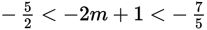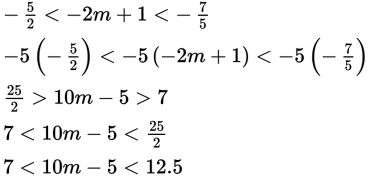# SAT Math Multiple Choice Question 821: Answer and Explanation

### Test Information

Question: 821

11. If, what is the greatest possible integer value of the expression 10m – 5?

• A. 6
• B. 7
• C. 10
• D. 12

Explanation:

D

Difficulty: Medium

Category: Heart of Algebra / Inequalities

Strategic Advice: You could solve the compound inequality for m and substitute the result into the expression 10m - 5, but there is a quicker way to answer a question like this. Look for a relationship between what you're given, the possible values of -2m + 1, and what you're looking for, the possible values of 10m - 5.

Getting to the Answer: Notice that 10m - 5 is -5 times the expression -2m + 1. This means you can answer the question by multiplying all three pieces of the inequality by -5. Don't forget to flip the inequality symbols because you are multiplying by a negative number. Then write the inequality with increasing values from left to right:The question asks for the greatest possible integer value, so the correct answer is 12, which is (D).